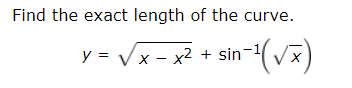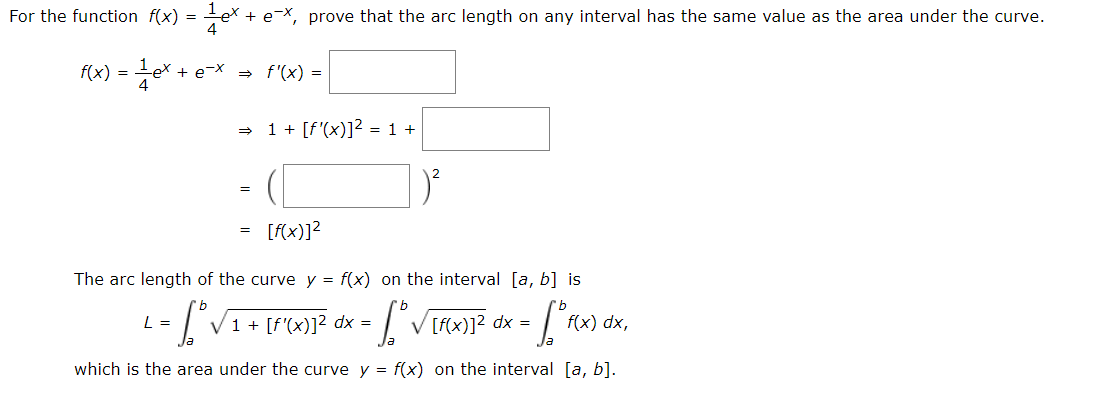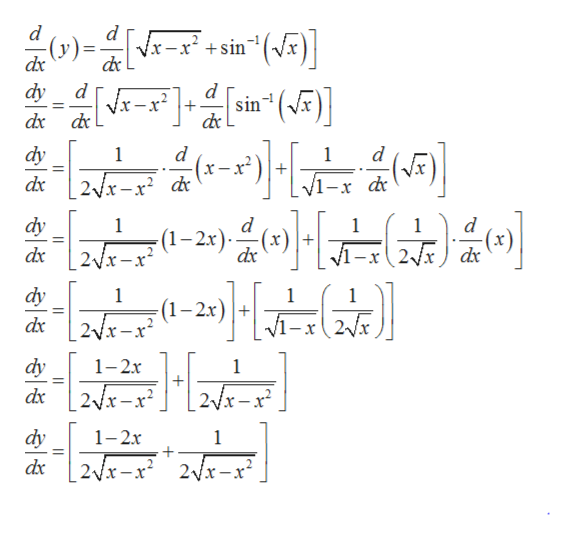# Find the exact length of the curvey = x-x2 +sin-1(V For the function f(x) = -e* + e *, prove that the arc length on any interval has the same value as the area under the curve.f(x) = e+ e~X f'(x) = |1[f'(x)]2 = 1=[f(x)]2The arc length of the curve y = f(x) on the interval [a, b] isfx)12 dx =L =f(x) dx,1 [f'(x)]2 dx =Vwhich is the area under the curve y f(x) on the interval [a, b]

Questionhelp_outlineImage TranscriptioncloseFind the exact length of the curve y = x-x2 +sin-1(V fullscreenhelp_outlineImage TranscriptioncloseFor the function f(x) = -e* + e *, prove that the arc length on any interval has the same value as the area under the curve. f(x) = e+ e~X f'(x) = | 1[f'(x)]2 = 1 = [f(x)]2 The arc length of the curve y = f(x) on the interval [a, b] is fx)12 dx = L = f(x) dx, 1 [f'(x)]2 dx = V which is the area under the curve y f(x) on the interval [a, b] fullscreen
check_circleExpert Solution
Step 1

Given:

Step 2

Formula used:

Step 3

Now to start, at first differentiating the given func...help_outlineImage Transcriptionclosed x-x'+sin"(Vx) dx dy d sin (x) Vx-x+ dx dy d x 1 1 x-x dc dy 1 (1-2x) 1 1 (x) 2Vx) dx dy 1 1 1 -2x 2x dy 1-2x 1 2Vx-x2 2/x-x dx dy 1- 1 dx 2x-x 2Vx-x fullscreen

### Want to see the full answer?

See Solution

#### Want to see this answer and more?

Solutions are written by subject experts who are available 24/7. Questions are typically answered within 1 hour*

See Solution
*Response times may vary by subject and question
Tagged in

### Derivative